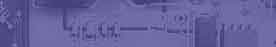Basic electrical laws and circuits analysis & circuits theory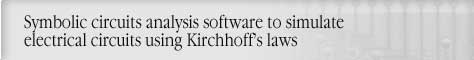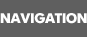HOMEDEMOSDOWNLOADORDEREE TUTORIALEE LINKSHOMEWORK HELPBasic electrical laws & circuits theory

Visit main page to learn more about Circuit Magic. (Symbolic electrical circuits simulation software to analyse circuits using Kirchhoff's Laws, Node Voltage and Mesh Currents .)

Direct currents circuits

Voltage, Current & Resistance
Direct currents devices
Ohm's Law
Superposition theorem
Kirchhoff's Current and Voltage Laws & circuit analysis sample
Norton & Thevenin theorem
Using Circuit Magic to find Norton & Thevenin equvalents
Resistors in Series & Resistors in Parallel
Kennelly's Star-Delta & Delta-Star Transformations
Using Circuit Magic to find any circuits resistances
Node Voltage Method description & circuit analysis sample
Mesh Current Method description & circuit analysis sample

Alternating currents circuits

Alternating Current (AC), Voltage, sinusoidal Waveform
Frequency, Period, Phase Angle of sinusoidal Waveform
Phasor (Vector) Diagram & Complex Numbers for AC Circuits Analysis(Java applet)
Alternating currents(AC) devices
Applying AC voltage to capacitor, coil, resistor(Java applet)
AC Circuits Power (Java applet)
Creating Phasor Diagrams with Circuit Magic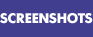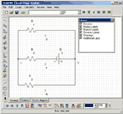Circuit editorAC Circuit more screenshots »